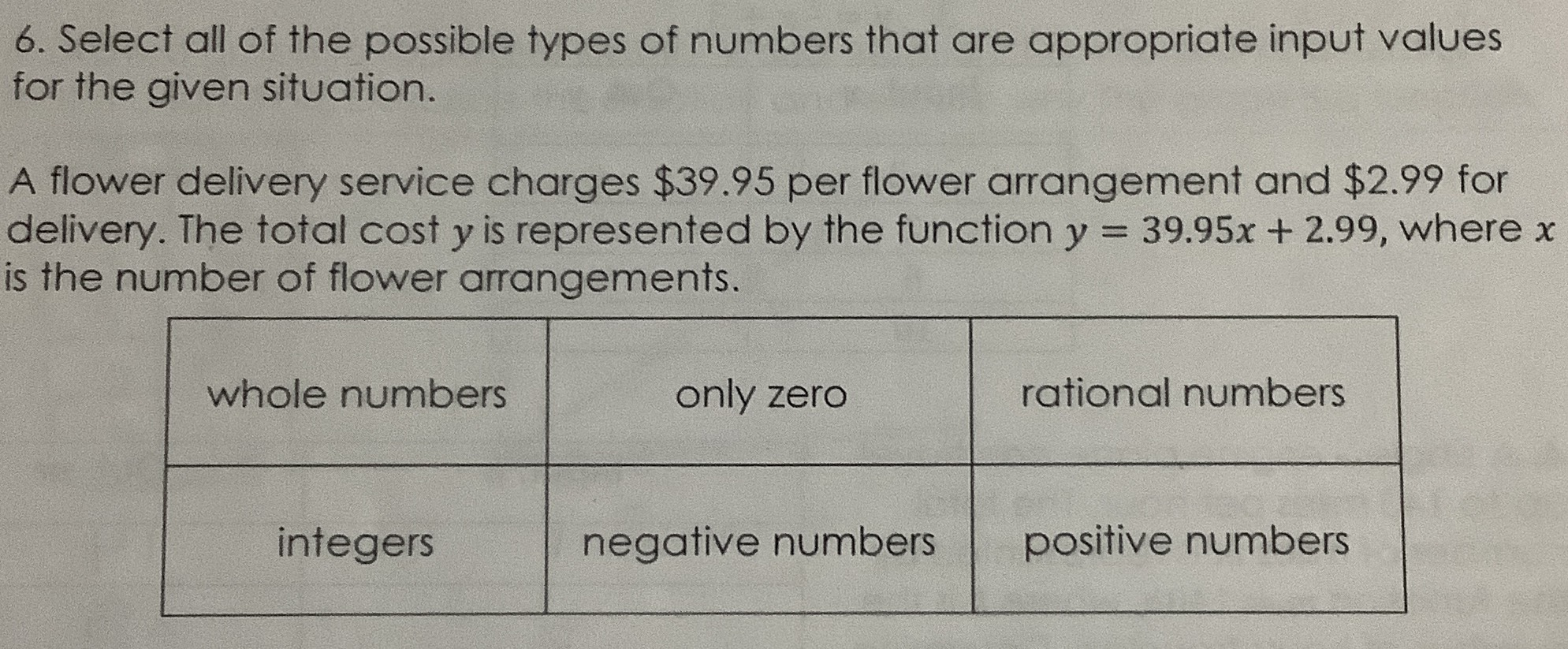### ¿Todavía tienes preguntas de matemáticas?

Pregunte a nuestros tutores expertos
Algebra
Pregunta6. Select all of the possible types of numbers that are appropriate input values for the given situation. A flower delivery service charges $$\ 39.95$$ per flower arrangement and $$\ 2.99$$ for delivery. The total cost $$y$$ is represented by the function $$y = 39.95 x + 2.99$$ , where $$x$$ is the number of flower arrangements.